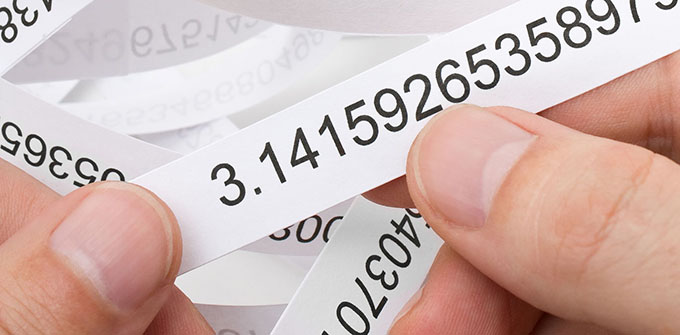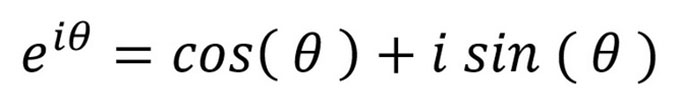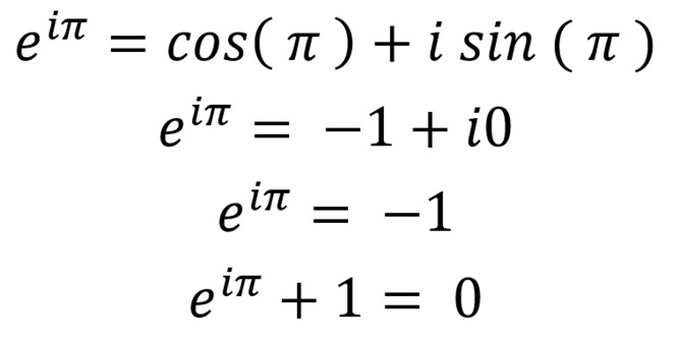# Pi and its part of the most beautiful formula in mathematics

Share:Pi Day is upon us again, for those who note today’s date in the format 3/14 (March 14). But rather than talk about Pi Day itself, as I did last year, this year I want to talk about Pi and mathematical notions of beauty.

How better to do so than to talk about the 18th century European scholar Leonard Euler’s famous formula:Euler’s beautiful formula. Note that e is the base for the natural logarithm and i is the symbol for the square root of -1, explained later.

Often described as “the most beautiful formula in mathematics”, Euler seems never to have actually written it down – naming conventions in mathematics are a bit dodgy. Rather, it is a special case of Euler’s discovery that exponential growth and circular motion are equivalent, given by the following formula:This formula is often referred to as cis, combining cos and sin together, and θ is the Greek symbol Theta.

The US theoretical physicist Richard Feynman called this “the most remarkable formula in mathematics”.

Ed Sandifer, a founder of the Euler Society, has a lovely 2007 article discussing in detail Euler’s approaches – over 40 years – to show how the formula (above) worked.

I shall try to get the story of this formula across with very few more symbols.

What the formula does

Euler’s formula involves five fundamental constants: 0, 1, i, e, and Pi, and on adding equality, addition and exponentiation, combines them into a seven-symbol word in a mysterious and useful way.

Equivalently, it can also be written:This is even more succinct and introduces negative numbers.

It is a common feature of mathematics that discoveries are often first used and only later understood. As the 18th century French mathematician Jean d’Alembert wrote: “algebra is generous, she often gives more than we ask”.

Let me discuss the 2,000-year history of the building blocks of Euler’s formula. You don’t have to understand the actual mathematics, just gain an appreciation of the origin of the various elements of the formula and how they combine so neatly together.

Equality (=)

The “=” symbol is attributed to the Welsh scientist Robert Recorde in 1557.

Arguments about the meaning of equality in mathematics have mirrored and driven discussions about definite descriptions in philosophy more generally.

The British logician Bertrand Russell’s famous example is Venus, described as the morning star and as the evening star. An over-discussed example in mathematics is whether 0.99999999… and 1 are equal. They are and they aren’t.

Zero (0)

Notions of nothingness and the void or infinity go back much further, but the Greeks and others had not discovered rules to manipulate with “0”.

A mathematically tractable notion of zero is attributed to the great Indian thinker Brahmagupta around 650CE.

When married with the other Indian discovery of positional notation, calculation became much more accessible. This ability did not come fully to Europe until the 15th century and later.

1

Without “1” there would be no advanced arithmetic. With “0” and “1”, we also have binary notation and modern digital computers. What the US theoretical physicist John Archibald Wheeler called “it from bit”.

This leads to modern group theory, algebra, cryptography and much, much else.

i

The use of imaginary numbers also dates largely from the 16th-17th century. The French philosopher and mathematician Rene Decartes used the term disparagingly.

Mathematical concepts we now take for granted sometimes took centuries to be adopted and understood. No wonder school children rebel.

It took Euler and then German mathematician Carl Friedrich Gauss to truly exploit imaginary numbers and make the word “imaginary” have a positive mathematical connotation.

Defining “i” as the square root of -1 has the wonderful consequence that a polynomial of degree n has n (complex) roots.

For example x4-1 = (x+1) (x-1) (x-i) (x+i), it has four roots. This leads to what is now called complex analysis.

Most of modern mathematics and mathematical physics (such as quantum theory) could not be done without complex analysis.

Pi (π)

Pi originates as the area of the circle of radius one or the circumference of a circle of diameter one.

The great Greek mathematician Archimedes of Syracuse (287-c212 BCE) used this idea to provide the approximation of 22/7 for Pi (3.141592…).

Euler discovered the modern definition which takes Pi/2 as the smallest positive zero of the cosine function defined by what is known as a Taylor series. This is a bit complicated but if you just think of the series as a very large polynomial you will get the idea.

Here n! = 1 x 2 x … x n is called the factorial of n. This was another 17th-century discovery.

e

The constant “e” originated in the 17th century as the base of the natural logarithm, and to three decimal places is 2.718…, though like Pi, it’s a transcendental number and continues without repeating to countless decimal places.

Euler, the master of us all – who named both “pi” and “e” – realised that ex also had a dandy Taylor series:

Then setting theta (θ) equal to one, gives an efficient formula for e.

Now we know all the building blocks all we need do in the second equation (above) is set Theta to be Pi, and with a little trigonometry, knowing that sin (π) = 0 and cos (π) = -1, then reducing the formula step by step, out pops the original beautiful formula.One Pi is entered into the formula and the calculations are made, a little mathematical juggling of various elements on either side of the = sign gives us the final beautiful formula.

What is mathematical beauty?

As you can see, to view the formula as beautiful it is necessary to understand the elements, at least roughly.

Bertrand Russell in his History of Western Philosophy
put it so:

Mathematics, rightly viewed, possesses not only truth, but supreme beauty – a beauty cold and austere, like that of sculpture, without appeal to any part of our weaker nature, without the gorgeous trappings of painting or music, yet sublimely pure, and capable of a stern perfection such as only the greatest art can show.

Most mathematicians would agree that to be beautiful a formula must be unexpected, concise and useful – in the rarefied sense that professional mathematicians recognise.

When forced to, most mathematicians will list Archimedes, Gauss and Euler among the top five mathematical thinkers of all time. The other two are Isaac Newton (for calculus and mechanics) and Bernhard Riemann (for the Riemann hypothesis and Riemannian geometry).

With three of these brilliant thinkers and fundamental constants engaged, it is no wonder that Euler’s formula is lionised as it is, as the most beautiful formula in mathematics.

Author Bio:Jonathan Borwein (Jon) is Laureate Professor of Mathematics at the University of Newcastle

Tags: